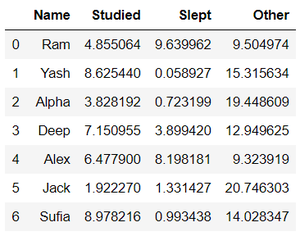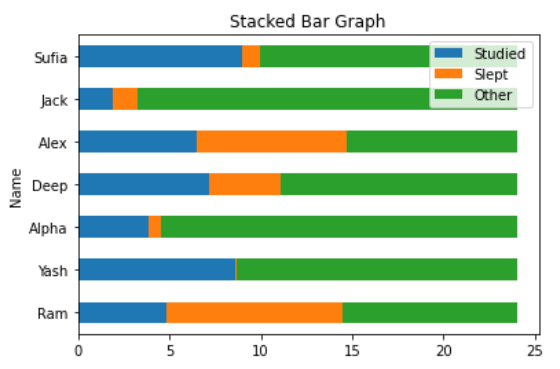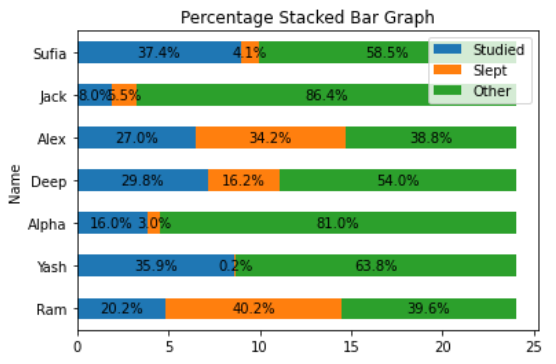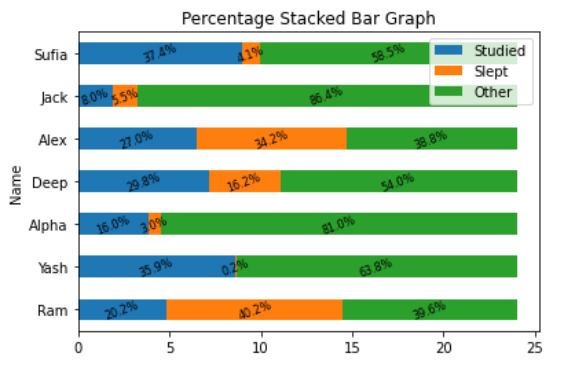Open in App
Not now

## Related Articles

• Write an Interview Experience

# Stacked Percentage Bar Plot In MatPlotLib

• Difficulty Level : Hard
• Last Updated : 01 Oct, 2020

A Stacked Percentage Bar Chart is a simple bar chart in the stacked form with a percentage of each subgroup in a group. Stacked bar plots represent different groups on the top of one another. The height of the bar depends on the resulting height of the combination of the results of the groups. It goes from the bottom to the value instead of going from zero to value. A percent stacked bar chart is almost the same as a stacked barchart. Subgroups are displayed on top of each other, but data are normalized to make in a sort that the sum of every subgroup is the same as the total for each one.

The dataset used in the following examples is shown below :Procedure: The procedure to draw Stacked Percentage Bar Chart is the following steps which are described below with examples :

1. Draw a stacked bar chart using data (dataset, dictionary, etc.).

## Python3

 `# importing packages``import` `pandas as pd``import` `matplotlib.pyplot as plt`` ` `# load dataset``df ``=` `pd.read_excel(``"Hours.xlsx"``)`` ` `# view dataset``print``(df)`` ` `# plot a Stacked Bar Chart using matplotlib``df.plot(``    ``x ``=` `'Name'``,``    ``kind ``=` `'barh'``,``    ``stacked ``=` `True``,``    ``title ``=` `'Stacked Bar Graph'``,``    ``mark_right ``=` `True``)`

Output:

```    Name   Studied     Slept      Other
0    Ram  4.855064  9.639962   9.504974
1   Yash  8.625440  0.058927  15.315634
2  Alpha  3.828192  0.723199  19.448609
3   Deep  7.150955  3.899420  12.949625
4   Alex  6.477900  8.198181   9.323919
5   Jack  1.922270  1.331427  20.746303
6  Sufia  8.978216  0.993438  14.028347

```2. Add Percentage on subgroups of each group.

## Python3

 `# importing packages``import` `pandas as pd``import` `numpy as np``import` `matplotlib.pyplot as plt`` ` `# load dataset``df ``=` `pd.read_excel(``"Hours.xlsx"``)`` ` `# view dataset``print``(df)`` ` `# plot a Stacked Bar Chart using matplotlib``df.plot(``  ``x ``=` `'Name'``, ``  ``kind ``=` `'barh'``, ``  ``stacked ``=` `True``, ``  ``title ``=` `'Percentage Stacked Bar Graph'``, ``  ``mark_right ``=` `True``)`` ` `df_total ``=` `df[``"Studied"``] ``+` `df[``"Slept"``] ``+` `df[``"Other"``]``df_rel ``=` `df[df.columns[``1``:]].div(df_total, ``0``)``*``100`` ` `for` `n ``in` `df_rel:``    ``for` `i, (cs, ab, pc) ``in` `enumerate``(``zip``(df.iloc[:, ``1``:].cumsum(``1``)[n], ``                                         ``df[n], df_rel[n])):``        ``plt.text(cs ``-` `ab ``/` `2``, i, ``str``(np.``round``(pc, ``1``)) ``+` `'%'``, ``                 ``va ``=` `'center'``, ha ``=` `'center'``)`

Output:

```     Name   Studied     Slept      Other
0    Ram  4.855064  9.639962   9.504974
1   Yash  8.625440  0.058927  15.315634
2  Alpha  3.828192  0.723199  19.448609
3   Deep  7.150955  3.899420  12.949625
4   Alex  6.477900  8.198181   9.323919
5   Jack  1.922270  1.331427  20.746303
6  Sufia  8.978216  0.993438  14.028347

```## Python3

 `# importing packages``import` `pandas as pd``import` `numpy as np``import` `matplotlib.pyplot as plt`` ` `# load dataset``df ``=` `pd.read_xlsx(``"Hours.xlsx"``)`` ` `# view dataset``print``(df)`` ` `# plot a Stacked Bar Chart using matplotlib``df.plot(``  ``x ``=` `'Name'``, ``  ``kind ``=` `'barh'``, ``  ``stacked ``=` `True``, ``  ``title ``=` `'Percentage Stacked Bar Graph'``, ``  ``mark_right ``=` `True``)`` ` `df_total ``=` `df[``"Studied"``] ``+` `df[``"Slept"``] ``+` `df[``"Other"``]``df_rel ``=` `df[df.columns[``1``:]].div(df_total, ``0``) ``*` `100`` ` `for` `n ``in` `df_rel:``    ``for` `i, (cs, ab, pc) ``in` `enumerate``(``zip``(df.iloc[:, ``1``:].cumsum(``1``)[n], ``                                         ``df[n], df_rel[n])):``        ``plt.text(cs ``-` `ab ``/` `2``, i, ``str``(np.``round``(pc, ``1``)) ``+` `'%'``, ``                 ``va ``=` `'center'``, ha ``=` `'center'``, rotation ``=` `20``, fontsize ``=` `8``)`

Output:

```    Name   Studied     Slept      Other
0    Ram  4.855064  9.639962   9.504974
1   Yash  8.625440  0.058927  15.315634
2  Alpha  3.828192  0.723199  19.448609
3   Deep  7.150955  3.899420  12.949625
4   Alex  6.477900  8.198181   9.323919
5   Jack  1.922270  1.331427  20.746303
6  Sufia  8.978216  0.993438  14.028347

```My Personal Notes arrow_drop_up